# Why 1 / ∞ = 0 but ∞ * 0 is not equal to 1?

• B
• danielassayag

#### danielassayag

As we know those relations are true: if a/b = c, then a = b*c and b = a/c

Therefore if 1/ ∞ = 0,

∞ * 0 should be equal to 1

and

1/0 = ∞

•russ_watters, member 428835 and sysprog
Why multiplying 0 by infinity should be zero and not one, when dividing 1 by infinity is 0?
the a/b = c equivalent to a = b*c relation doesn't apply here?

Last edited:
Division by zero or by infinity is undefined because they lead to mathematical inconsistencies as you have discovered.
Division by infinity is not undefined. Division by infinity tends to zero, The plot is stretched oppositely whether the number is smaller or greater than one.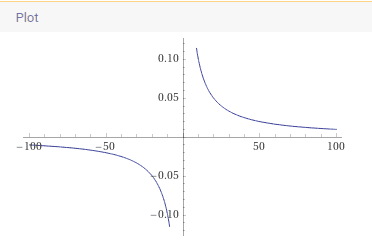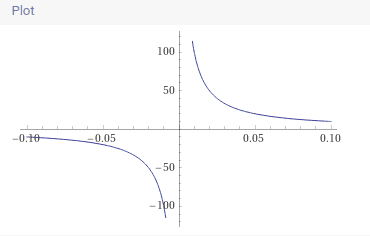plot | 1/x | x = -0.1 to 0.1
plot 1/x from x=-100 to 100

•weirdoguy
Division by infinity is not undefined.
You can say that over and over as many times as you like, but saying it is not going to make it true.

•russ_watters, member 428835 and jedishrfu
You can say that over and over as many times as you like, but saying it is not going to make it true.
You are right. I just realized that 1/x when x gets larger tends to 0.
But if x is infinitely smaller, example 1/0.1, 1/0.01.., then it tends to infinity again.
So 1/infinity tends both to zero and infinity. So i guess you can call it undefined.

You can say that over and over as many times as you like, but saying it is not going to make it true.
I didn't mean to outsmart wolframalpha btw..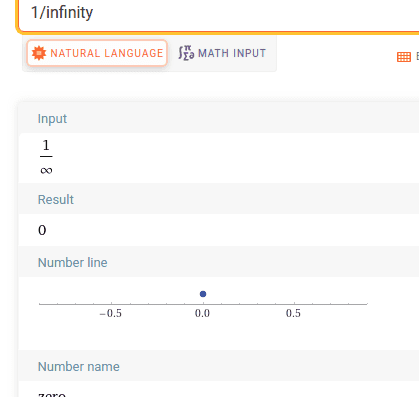So i guess you can call it undefined.
It's nice that you are now in sync with the rest of the world.

The problem with infinity is it is not a number, it is a label for the furthest point on the number line named so we can talk about not so we can do arithmetic with it.

As you noted, zero times any number is 0 and a number times its reciprocal is 1 but when using infinity as a number you get confusing ambiguous answers and so mathematicians declare it to be undefined.

•russ_watters
It's nice that you are now in sync with the rest of the world.
Wolfram alpha and microsoft don't seem to implement your understanding of maths, as they don't comprehend that 1/infinity should be undefined. Though they understand the concept of undefined itself, when it comes to dividing 1/0.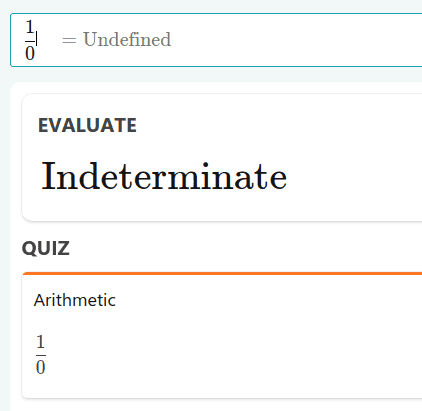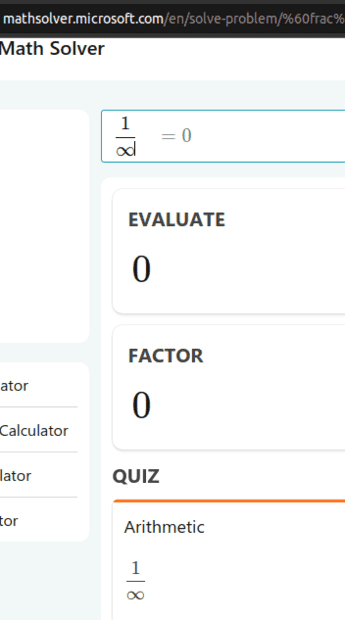You are right. I just realized that 1/x when x gets larger tends to 0.
And in mathematics symbols we write this: $$\lim_{x \to \infty}\frac 1 x = 0$$
But if x is infinitely smaller, example 1/0.1, 1/0.01.., then it tends to infinity again.
But what if x approaches zero from the negative side? Then the result gets unboundedly negative.
In terms of limits,
$$\lim_{x \to 0^-}\frac 1 x = -\infty$$
$$\lim_{x \to 0^+}\frac 1 x = +\infty$$
For this reason, ##\frac 1 0## is undefined, notwithstanding what Wolframalpha seems to indicate. In fact, division of any number by zero is also undefined.
So 1/infinity tends both to zero and infinity. So i guess you can call it undefined.
Absolutely not, and this makes no sense. The symbol ##\infty## is not a number in the real number system, and cannot be used in expression involving arithmetic or algebraic operations. Note that the Extended Reals do include ##\infty##, however.

•danielassayag
Why multiplying 0 by infinity should be zero and not one, when dividing 1 by infinity is 0?
the a/b = c equivalent to a = b*c relation doesn't apply here?
Because ##\infty## is not a number in the real number system, so multiplication of it is not defined.
##\frac a b = c \Leftrightarrow a = b \cdot c## provided that ##b \ne 0##. You need to read the fine print.

•Klystron
I think some of the above comments are unnecessarily dogmatic. There are definitely contexts where it makes sense to write ##1/\infty=0.## My issue with the logic in the OP is that you end up with the quotient ##\infty/\infty##, which is definitely undefined- you can't just say that it is ##1.##

Last edited:
••weirdoguy and jedishrfu
in some case, (when x get larger and larger) 1/x tends to zero, but only tends, which mean it's not completely 0, there is still some infinitely small value left to it. If you multiply that infinitely small value of 0 decimal, to an infinitely large integer number, you end multiplying the depth of decimal against the depth of integer, which is equal ; therefore you get back you an equality, 1.

1/infinity tends to zero, but only tends, which mean it's not completely 0, there is still some infinitely small value left to it.
This isn't true. A function may tend towards a limit, but a simple quotient doesn't. Even if you want to interpret ##1/\infty## as a limit, limits still equal their value. For example, ##\lim_{x\to\infty}1/x## equals zero; it doesn't "tend to zero" (though the function ##x\mapsto 1/x## does in the limit of large x.)

If you multiply that infinitely small value of 0 decimal, to an infinitely large integer number, you end multiplying the depth of decimal against the depth of integer, which is equal ; therefore you get back you an equality, 1.
This doesn't follow.

Wolfram alpha and microsoft don't seem to implement your understanding of maths, as they don't comprehend that 1/infinity should be undefined.
That's their problem, not mine.

••DaveE, QHDX666, jbriggs444 and 2 others
•weirdoguy and phinds
That's their problem, not mine.
I don't think it's a "problem" of theirs. There are lots of contexts in which ##1/\infty## is defined to be zero and it makes sense to me for it to be implemented that way.

•DaveE and ergospherical
You quoted this:
danielassayag said:
1/infinity tends to zero, but only tends, which mean it's not completely 0, there is still some infinitely small value left to it.
to then respond:
This isn't true. A function may tend towards a limit, but a simple quotient doesn't.
but here is what I wrote: (as you can see it hasnt been edited on the post)
in some case, (when x get larger and larger) 1/x tends to zero, but only tends, which mean it's not completely 0, there is still some infinitely small value left to it.
I'm interpreting 1/x with x getting infinitely larger and larger as a function, that when x gets larger, it gets closer and closer to 0.
I realize that 1/x (with x getting infinitely smaller and smaller than 1) tends to a greater and greater number.

Wolfram alpha and microsoft don't seem to implement your understanding of maths, as they don't comprehend that 1/infinity should be undefined. Though they understand the concept of undefined itself, when it comes to dividing 1/0.

View attachment 294454 View attachment 294455
View attachment 294456
Pretty simple to demonstrate and Wolfram agrees.

First, what does Wolfram says about ##0 \times \infty##: undefined

But Wolfram also says that ##\frac{1}{\infty} = 0##. It is not undefined. So why isn't ##0 \times \infty = 1##?

Because Wolfram also says that:
This is why ##0 \times \infty## is undefined: it can lead to an infinite number of answers. ##1## is just one of them.

The same problem arises with ##\frac{\infty}{\infty}## which is also undefined even though ##1 \times\infty = \infty##. That's because ##a \times \infty = \infty## no matter the value of ##a##.

Another way to look at it is that if we multiply anything by ##0##, it gives ##0##, and anything multiplied by ##\infty## equals ##\infty##. What if we multiplied ##0 \times \infty##? It is undefined because it could at least be either ##0## or ##\infty## (or anything else as demonstrated previously).

•DaveE, mathwonk and jbergman
There was a little comment in baby Rudin saying that it is conventional, in the extended real number system ##\mathbf{R} \cup \{ +\infty, -\infty \}##, to put ##x + \infty = +\infty##, and ##x - \infty = -\infty##, and ##\frac{x}{+\infty} = \frac{x}{-\infty} = 0##. The result of multiplying ##x\neq 0## by ##\pm \infty## is defined, similarly, as per common sense.

•Infrared
There are definitely contexts where it makes sense to write 1/∞=0.
But mathematics (excluding the Extended Reals) isn't one of those contexts.
My issue with the logic in the OP is that you end up with the quotient ∞/∞, which is definitely undefined- you can't just say that it is
More to the point, this is an indeterminate form, often written in mathematics textbooks as ##\left[\frac \infty \infty \right]##. The usual context for indeterminate forms is in limits such as the following.
$$\lim_{x \to \infty} \frac {3x} x = 3$$
$$\lim_{x \to \infty} \frac {x} {3x} = \frac 1 3$$
$$\lim_{x \to \infty} \frac {x} {x^2} = 0$$
In all three examples, both the numerator and denominator are increasing without bound, but the resulting limits are all different.

I don't think it's a "problem" of theirs. There are lots of contexts in which ##1/\infty## is defined to be zero and it makes sense to me for it to be implemented that way.
I remember a student who was asked to give an example of an unbounded sequence and his answer was:$$1,2,3, \infty, 4, 5 \dots$$

•Infrared
Pretty simple to demonstrate and Wolfram agrees.

First, what does Wolfram says about 0×∞: undefined

But Wolfram also says that ##\frac{1}{\infty} = 0##. It is not undefined. So why isn't 0×∞=1?
Because wolframalpha is inconsistent.

This is why 0×∞ is undefined: it can lead to an infinite number of answers.
An expression that can lead to multiple answers is called an indeterminate form. Other such forms include ##[\infty \pm \infty], [1^\infty], [\frac 0 0]## and others.

But mathematics (excluding the Extended Reals) isn't one of those contexts.

Of course there are mathematical contexts where it makes sense! The one that first comes to my mind is that the Riemann sphere ##\hat{\mathbb{C}}=\mathbb{C}\cup\{\infty\}## has the natural structure of a complex curve, and its automorphism group is the group of Mobius transformations ##z\mapsto\frac{az+b}{cz+d},## but in order for this to make sense on the whole sphere, you need to set ##1/\infty=0## and ##1/0=\infty.##

Because wolframalpha is inconsistent.
It's not inconsistent: ##0\cdot\infty## is indeterminate, but ##1/\infty## is not.

As an aside, I can think of at least case where ##0\cdot\infty## is sometimes defined: in measure/integration theory it is in fact usually set to 0 because integrating a function which is (almost) always zero on an infinitely large set, or integrating an infinitely large function on a set of measure zero give zero. But it's still indeterminate...

Of course there are mathematical contexts where it makes sense! The one that first comes to my mind is that the Riemann sphere ##\hat{\mathbb{C}}=\mathbb{C}\cup\{\infty\}## has the natural structure of a complex curve, and its automorphism group is the group of Mobius transformations ##z\mapsto\frac{az+b}{cz+d},## but in order for this to make sense on the whole sphere, you need to set ##1/\infty=0## and ##1/0=\infty.##
I explicitly excluded the extended reals.
Because wolframalpha is inconsistent.
It's not inconsistent: ##0\cdot\infty## is indeterminate, but ##1/\infty## is not.
But WA reports that ##\frac 1 \infty## is a number, and outside of the extended reals, it's not legitimate to use the symbol ##\infty## in arithmetic expressions.

I explicitly excluded the extended reals.
The Riemann sphere is not the extended reals.

But WA reports that ##\frac 1 \infty## is a number, and outside of the extended reals, it's not legitimate to use the symbol ##\infty## in arithmetic expressions.
It depends on what arithmetic you're doing. I agree it's not an equality of real numbers, but I think in any context where you can do arithmetic with ##\infty##, then ##1/\infty## will be zero.

Infrared said:
Riemann sphere ##\hat{\mathbb{C}}=\mathbb{C}\cup\{\infty\}##

The Riemann sphere is not the extended reals.
Tomato, tomahto -- you've extended the complex numbers to include ##\infty##.

Tomato, tomahto -- you've extended the complex numbers to include ##\infty##.
They're very different. The Riemann sphere only has one infinity; the extended reals has two. The Riemann sphere has the natural structure of a complex curve, which is why the Mobius transformations are applicable. This isn't some obscure object: it's the most basic example of a Riemann surface.

And why are we excluding the extended reals in the first place?

And why are we excluding the extended reals in the first place?
Because in the ordinary reals, the symbol ##\infty## does not represent a number, so expressions such as ##\frac 1 \infty## are not defined.

Given that this thread is marked 'B', discussions about the extended reals and the Riemann sphere are way off topic.

•DaveE and PeroK
Given that this thread is marked 'B', discussions about the extended reals and the Riemann sphere are way off topic.

This could well be the case, but then I don't think it's fair to say that wolfram alpha is wrong or inconsistent. It doesn't know about the limited usage which may or may not be relevant here.

I don't plan to message more on this issue, for fear of further derailing the thread.

I think a natural way to see the problem is to notice that ##2/\infty =0##, so ##\infty \times 0=2##. You can pick any number in the numerator here. So the multiplication clearly doesn't make sense even if the original equation has meaning to it. It doesn't matter what definition you try to attach to these operations

•DaveE, mathwonk and PeroK
Just to add to the fun, there are contexts in which ##\infty \times 0 = 0##.

Just to add to the fun, there are contexts in which ##\infty \times 0 = 0##.
I think I mentioned this already in post 25 :)

•martinbn
1/∞ is not zero, it tends towards zero because the difference between numerator and denominator is huge, it certainly is undefined in common mathematics, however in certain cases such as in physics it is taken as zero because the difference is huge and we are not aiming for much precision in physics; eg:- NASA uses only till 15 digits of pi after decimal point for some of its highest accuracy calculations.

•PeroK